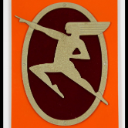# getting geodesic area -ve

241
6
05-22-2020 08:20 AMby
Occasional Contributor III

i am using below function to calculate area every time i add and delete a new polygon, It was working fine, but suddenly, i need to change the third party layer which provides polygon vertices for property and now i receive my area in negative value. so in order to co up with it, I added -ve in front of it,

But when i draw a polygon by hand using sketch view model, then i get area in +ve and my area calculated reduces for below formu;la instead of increasing.

Could anyone help me with this??

//Calculate TOtal hectares of Map Geometry
function calculateArea() {
var area = 0;
setTimeout(function() {
graphicsLayer.graphics.map(function (grap) {
area = area+(-(geometryEngine.geodesicArea(grap.geometry, "hectares")));
});
totalAreaHectare=Math.round(area);
jQuery("#hectare").html('<b>'+totalAreaHectare+' HECTARES</b>');
}, 500);
} //End calculateArea

1 Solution

Accepted SolutionsbyMVP Honored Contributor

Are you just wishing to get the correct area or are you doing other things with those geometries?

Why aren't you working with the geometry itself?

``````graphicsLayer.graphics.map(function (grap) {
const simplified = geometryEngine.simplify(grap.geometry);
...
}
‍‍‍‍‍``````

If timing is an issue, you can always work with the asynchronous geometry engine

6 RepliesbyMVP Honored Contributor

If a polygon is drawn counter clockwise, then the area will be a negative number (they are considered to be holes). You can check this with the isClockwise methodby
Occasional Contributor III

Sir i get the vertices of polygon from linz db, how can i draw them counter clockwisebyMVP Honored Contributor

Try using the simplify method of the Geometry Engineby
Occasional Contributor III

I tried it like this, but it stop to display geometry other dom elements on my map

var newvertices=geometryEngine.simplify(vertices);
const polygon = createGeometry(newvertices);byMVP Honored Contributor

Are you just wishing to get the correct area or are you doing other things with those geometries?

Why aren't you working with the geometry itself?

``````graphicsLayer.graphics.map(function (grap) {
const simplified = geometryEngine.simplify(grap.geometry);
...
}
‍‍‍‍‍``````

If timing is an issue, you can always work with the asynchronous geometry engineby
Occasional Contributor III

Thanks ken It worked , i just need to calculate exact  area